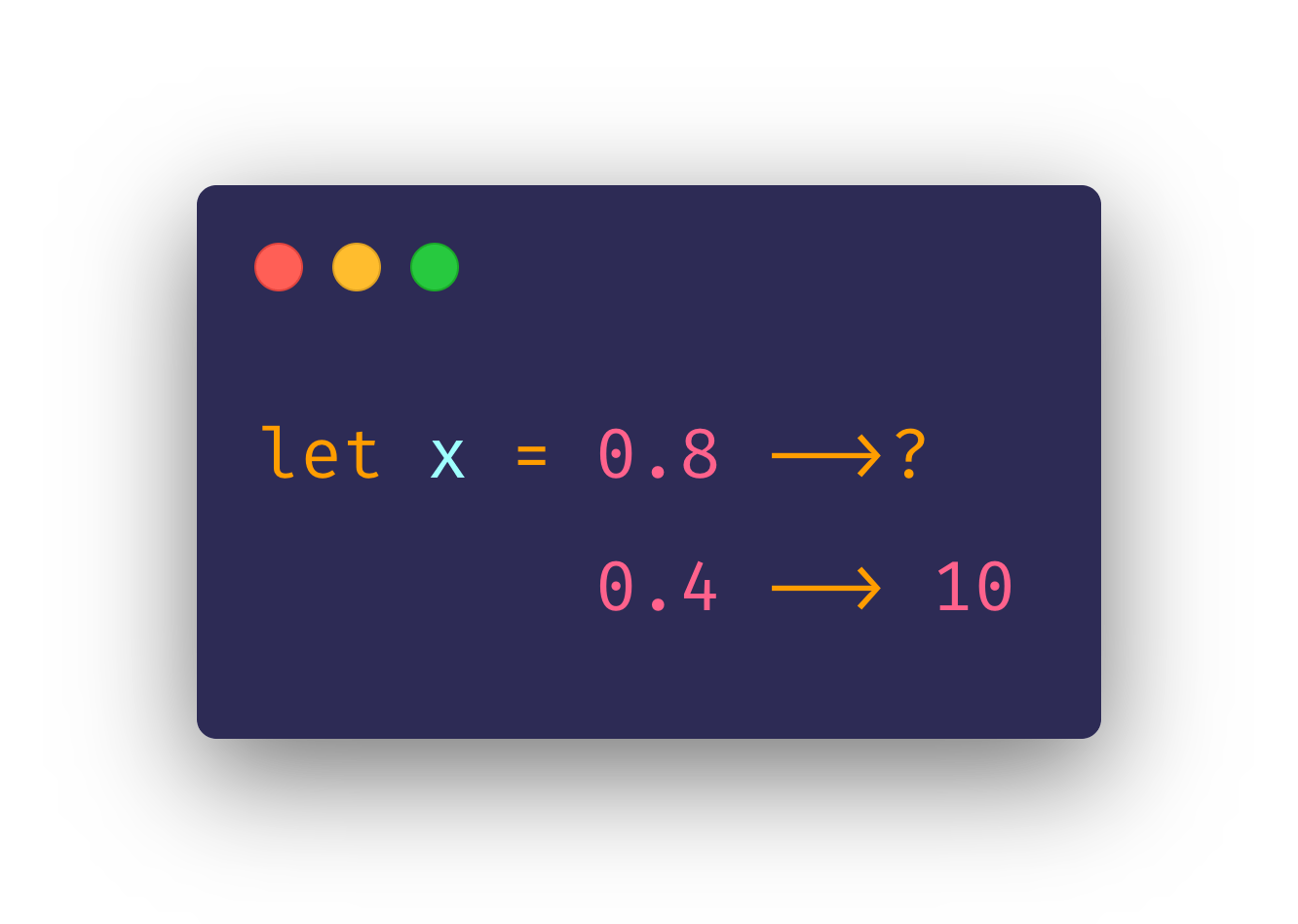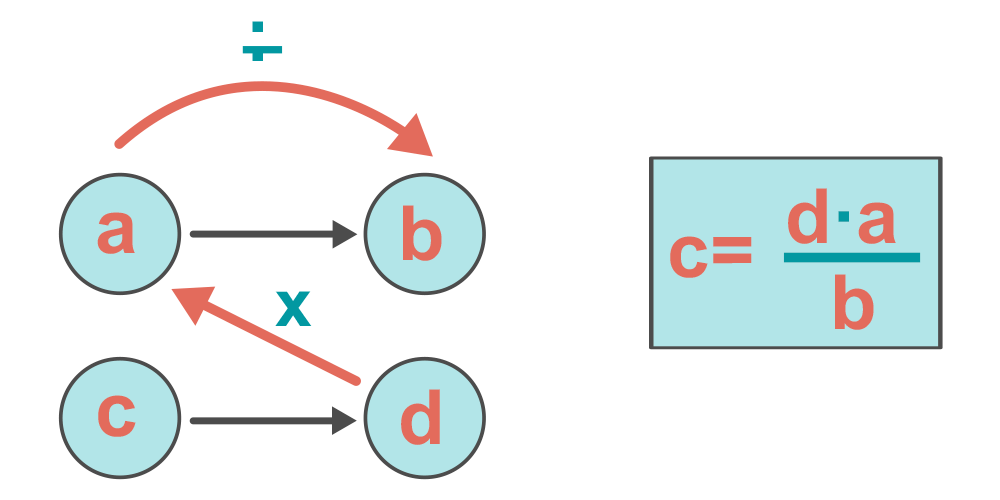# Swift Custom Operator — The Rule of 3

### A throwback to Elementary Math Classes 🤓I always had this idea of creating something like this in a language, since it is probably the most overused math operation in every application. I remember in my math class days, where we would draw this graph on paper in order to calculate the unknown datum in a proportional problem.So how is this possible to create in Swift? First, to clear things out, the code above looks like that with some code formatting and with the Fira Code font. Without any of those, the code would look like this:

`let x = 0.8 -->? 0.4 --> 10`

As you can see, the formatting and the question mark are useful to understand what value we are calculating, and it is noticeable that this is actually not one custom operator, but two custom operators that form a math operation. The symbol `-->` has been used since `->` is a reserved symbol in Swift.

So, my first thought was to create a custom ternary operator, but at least for now, this is not possible in swift. What we can do is create two custom operators, the `-->` is pretty simple, it just tuples the left and right value, returning a `(Double, Double)`. The `-->?` operator is the one that will perform the math calculation since it has all values. It receives a`Double`value on the left and on the right a closure function that returns a tuple `() -> (Double, Double)` which is exactly what the `-->` operator is.

``````infix operator -->?: TernaryPrecedence

func -->?(lhs: Double,
rhs: @escaping @autoclosure () -> (Double, Double)
) -> Double {
return lhs * rhs().1 / rhs().0
}

infix operator -->

func -->(lhs: Double, rhs: Double) -> (Double, Double) {
return (lhs, rhs)
}

let x = 0.8 -->?
0.4 --> 10``````

## Let’s break it down

### →

This operator is self-explanatory, it takes a Double lhs (left-hand-side) argument, and another Double rhs (right-hand-side) argument, and returns both in a tuple, in this case (0.4, 10). It is an infix operator since it operates in two targets, so that's why we have the left and right side arguments. And since we don’t specify the Precedence Group, it has the Default one, which has no associativity, since we are not interested in chaining multiple operations.

### →?

This is where all the magic happens 🧙‍♂️✨. It is also an infix operator, where on the lhs argument, we have the value that we want to know in the unknown proportion, and on the rhs we receive a function as an argument, which is the `-->` operation function wrapped in a closure so we can call it later to give us the tuple with the known proportional values. The `@autoclosure` keyword is important to let us write a closure without the curly brackets, or the final expression would look like this instead: `let x = 0.8 -->? { 0.4 -> 10 }` . The TerneraryPrecedence has a right-associativity, which is what we want, so the right expression is evaluated first.

## Conclusion

Swift let us be very creative with Custom Operators, it was indeed really fun to create this one, but of course, we need to be careful when deciding using a customer operator in our codebase, especially when we work in a team of multiple developers. We want to make our codebase as simple as possible, and introducing custom operators will for sure be harder to onboard newcomers. Unless we are building a DSL for a very specific problem, or if it’s a custom operator that will clearly improve the codebase in a specific domain, I would stay away from overusing Custom Operators.